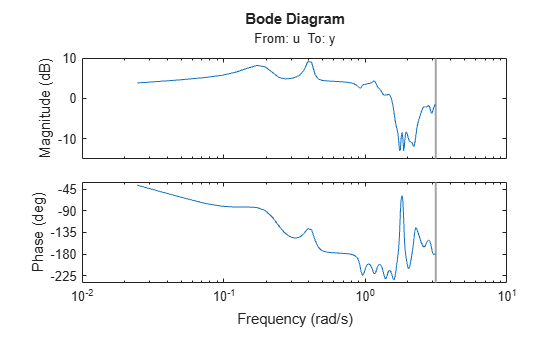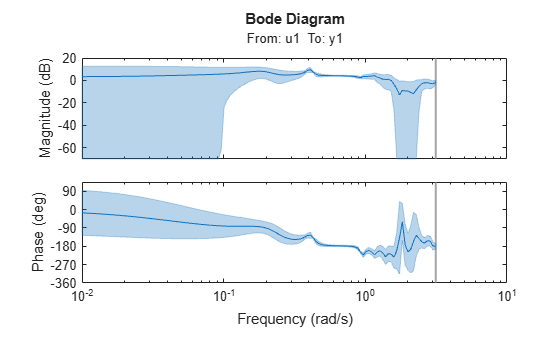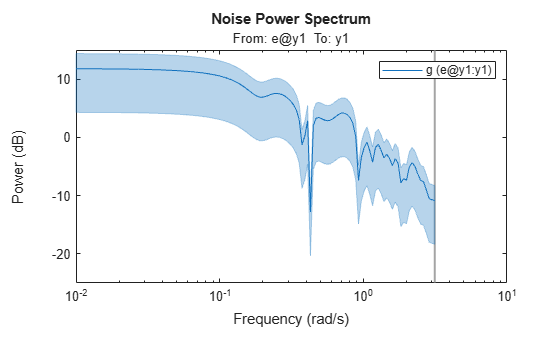# spa

Estimate frequency response with fixed frequency resolution using spectral analysis

## Syntax

```G = spa(data) G = spa(data,winSize,freq) G = spa(data,winSize,freq,MaxSize) ```

## Description

`G = spa(data)` estimates frequency response (with uncertainty) and noise spectrum from time- or frequency-domain data. `data` is an `iddata` or `idfrd` object and can be complex valued. `G` is as an `idfrd` object. For time-series `data`, `G` is the estimated spectrum and standard deviation.

Information about the estimation results and options used is stored in the model's `Report` property. `Report` has the following fields:

• `Status` — Summary of the model status, which indicates whether the model was created by construction or obtained by estimation.

• `Method` — Estimation command used.

• `WindowSize` — Size of the Hann window.

• `DataUsed` — Attributes of the data used for estimation. Structure with the following fields:

• `Name` — Name of the data set.

• `Type` — Data type.

• `Length` — Number of data samples.

• `Ts` — Sample time.

• `InterSample` — Input intersample behavior.

• `InputOffset` — Offset removed from time-domain input data during estimation.

• `OutputOffset` — Offset removed from time-domain output data during estimation.

`G = spa(data,winSize,freq)` estimates frequency response at frequencies `freq`. `freq` is a row vector of values in rad/`TimeUnit`, where `TimeUnit` refers to the `TimeUnit` property of data. For discrete-time data, set `freq` within the Nyquist frequency bound. `winSize` is a scalar integer that sets the size of the Hann window.

`G = spa(data,winSize,freq,MaxSize)` can improve computational performance using `MaxSize` to split the input-output data such that each segment contains fewer than `MaxSize` elements. `MaxSize` is a positive integer.

## Examples

collapse all

Estimate frequency response with fixed resolution at 128 equally spaced, logarithmic frequency values between 0 (excluded) and $\pi$.

```load iddata3; g = spa(z3); bode(g)```Define the frequency vector.

`w = logspace(-2,pi,128);`

Compute the frequency response.

```load iddata3; g = spa(z3,[],w);```

`[]` specifies the default lag window size.

Plot the Bode response and disturbance spectrum with confidence interval of 3 standard deviations.

```h = bodeplot(g); showConfidence(h,3)``````figure h = spectrumplot(g); showConfidence(h,3)```collapse all

### Frequency Response Function

Frequency response function describes the steady-state response of a system to sinusoidal inputs. For a linear system, a sinusoidal input of a specific frequency results in an output that is also a sinusoid with the same frequency, but with a different amplitude and phase. The frequency response function describes the amplitude change and phase shift as a function of frequency.

To better understand the frequency response function, consider the following description of a linear, dynamic system:

`$y\left(t\right)=G\left(q\right)u\left(t\right)+v\left(t\right)$`

where u(t) and y(t) are the input and output signals, respectively. G(q) is called the transfer function of the system—it captures the system dynamics that take the input to the output. The notation G(q)u(t) represents the following operation:

`$G\left(q\right)u\left(t\right)=\sum _{k=1}^{\infty }g\left(k\right)u\left(t-k\right)$`

q is the shift operator, defined by the following equation:

G(q) is the frequency-response function, which is evaluated on the unit circle, G(q=eiw).

Together, G(q=eiw) and the output noise spectrum ${\stackrel{^}{\Phi }}_{v}\left(\omega \right)$ are the frequency-domain description of the system.

The frequency-response function estimated using the Blackman-Tukey approach is given by the following equation:

`${\stackrel{^}{G}}_{N}\left({e}^{i\omega }\right)=\frac{{\stackrel{^}{\Phi }}_{yu}\left(\omega \right)}{{\stackrel{^}{\Phi }}_{u}\left(\omega \right)}$`

In this case, ^ represents approximate quantities. For a derivation of this equation, see the chapter on nonparametric time- and frequency-domain methods in System Identification: Theory for the User, Second Edition, by Lennart Ljung, Prentice Hall PTR, 1999.

### Output Noise Spectrum

The output noise spectrum (spectrum of v(t)) is given by the following equation:

`${\stackrel{^}{\Phi }}_{v}\left(\omega \right)={\stackrel{^}{\Phi }}_{y}\left(\omega \right)-\frac{{|{\stackrel{^}{\Phi }}_{yu}\left(\omega \right)|}^{2}}{{\stackrel{^}{\Phi }}_{u}\left(\omega \right)}$`

This equation for the noise spectrum is derived by assuming the linear relationship $y\left(t\right)=G\left(q\right)u\left(t\right)+v\left(t\right)$, that u(t) is independent of v(t), and the following relationships between the spectra:

`${\Phi }_{y}\left(\omega \right)={|G\left({e}^{i\omega }\right)|}^{2}{\Phi }_{u}\left(\omega \right)+{\Phi }_{v}\left(\omega \right)$`
`${\Phi }_{yu}\left(\omega \right)=G\left({e}^{i\omega }\right){\Phi }_{u}\left(\omega \right)$`

where the noise spectrum is given by the following equation:

`${\Phi }_{v}\left(\omega \right)\equiv \sum _{\tau =-\infty }^{\infty }{R}_{v}\left(\tau \right){e}^{-iw\tau }$`

${\stackrel{^}{\Phi }}_{yu}\left(\omega \right)$ is the output-input cross-spectrum and ${\stackrel{^}{\Phi }}_{u}\left(\omega \right)$ is the input spectrum.

Alternatively, the disturbance v(t) can be described as filtered white noise:

`$v\left(t\right)=H\left(q\right)e\left(t\right)$`

where e(t) is the white noise with variance $\lambda$ and the noise power spectrum is given by the following equation:

`${\Phi }_{v}\left(\omega \right)=\lambda {|H\left({e}^{i\omega }\right)|}^{2}$`

## Algorithms

`spa` applies the Blackman-Tukey spectral analysis method by following these steps:

1. Computes the covariances and cross-covariance from u(t) and y(t):

`$\begin{array}{l}{\stackrel{^}{R}}_{y}\left(\tau \right)=\frac{1}{N}\sum _{t=1}^{N}y\left(t+\tau \right)y\left(t\right)\\ {\stackrel{^}{R}}_{u}\left(\tau \right)=\frac{1}{N}\sum _{t=1}^{N}u\left(t+\tau \right)u\left(t\right)\\ {\stackrel{^}{R}}_{yu}\left(\tau \right)=\frac{1}{N}\sum _{t=1}^{N}y\left(t+\tau \right)u\left(t\right)\end{array}$`
2. Computes the Fourier transforms of the covariances and the cross-covariance:

`$\begin{array}{l}{\stackrel{^}{\Phi }}_{y}\left(\omega \right)=\sum _{\tau =-M}^{M}{\stackrel{^}{R}}_{y}\left(\tau \right){W}_{M}\left(\tau \right){e}^{-i\omega \tau }\\ {\stackrel{^}{\Phi }}_{u}\left(\omega \right)=\sum _{\tau =-M}^{M}{\stackrel{^}{R}}_{u}\left(\tau \right){W}_{M}\left(\tau \right){e}^{-i\omega \tau }\\ {\stackrel{^}{\Phi }}_{yu}\left(\omega \right)=\sum _{\tau =-M}^{M}{\stackrel{^}{R}}_{yu}\left(\tau \right){W}_{M}\left(\tau \right){e}^{-i\omega \tau }\end{array}$`

where ${W}_{M}\left(\tau \right)$ is the Hann window with a width (lag size) of M. You can specify `M` to control the frequency resolution of the estimate, which is approximately equal 2π/`M` rad/sample time.

By default, this operation uses 128 equally spaced frequency values between 0 (excluded) and π, where ```w = [1:128]/128*pi/Ts``` and `Ts` is the sample time of that data set. The default lag size of the Hann window is ```M = min(length(data)/10,30)```. For default frequencies, uses fast Fourier transforms (FFT)—which is more efficient than for user-defined frequencies.

### Note

`M =`γ is in Table 6.1 of Ljung (1999). Standard deviations are on pages 184 and 188 in Ljung (1999).

3. Compute the frequency-response function ${\stackrel{^}{G}}_{N}\left({e}^{i\omega }\right)$ and the output noise spectrum ${\stackrel{^}{\Phi }}_{v}\left(\omega \right)$.

`${\stackrel{^}{G}}_{N}\left({e}^{i\omega }\right)=\frac{{\stackrel{^}{\Phi }}_{yu}\left(\omega \right)}{{\stackrel{^}{\Phi }}_{u}\left(\omega \right)}$`
`${\Phi }_{v}\left(\omega \right)\equiv \sum _{\tau =-\infty }^{\infty }{R}_{v}\left(\tau \right){e}^{-iw\tau }$`

`spectrum` is the spectrum matrix for both the output and the input channels. That is, if `z = [data.OutputData`, `data.InputData]`, `spectrum` contains as spectrum data the matrix-valued power spectrum of `z`.

`$S=\sum _{m=-M}^{M}Ez\left(t+m\right)z{\left(t\right)}^{\prime }{W}_{M}\left({T}_{s}\right)\mathrm{exp}\left(-i\omega m\right)$`

`'` is a complex-conjugate transpose.

## References

Ljung, L. System Identification: Theory for the User, Second Ed., Prentice Hall PTR, 1999.